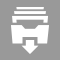#FilesDocument 73k

PercentBoard is a math game, in which you try to get the high score, using your knowledge of percentages and pre-algebra. Roll the dice,...Document 71k

NumberBoard is a math game, in which you try to get the high score, using your knowledge of arithmetic and pre-algebra. Roll the dice, move...Document 14k

How a small group of Spanish fortune hunters overthrew the powerful Aztec empire is one of history's most fascinating tales. This study...Document 21k

This is the first installation of a Middle School Debate Curriculum.  It covers definitions, introductions, and an overview to implementing...Presentation 335k

THIS FILE CONTAINS A COMMON CORE ALGEBRA 1 EXAMPLE OF PROCEDURES FOR SOLVING A QUADRATIC EQUATION USING FACTORING, QUADRATIC EQUATION, AND...Presentation 121k

THIS FILE CONTAINS AN EXAMPLE OF A COMMON CORE ALGEBRA 1 QUESTION OF DETERMINING AN EQUATION OF A GRAPH OF AN EXPONENTIAL EQUATION.  IT...Presentation 141k

THIS FILE CONTAINS AN EXAMPLE OF A COMMON CORE ALGEBRA TEST OF AN EXPLANATION OF AN EXAMPLE OF GRAPHING OVER INTERVALS. IT IS FORMATTED...

## Stuck?

Find the perfect tutor and raise your grades.